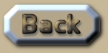Simulation of Jupiter's orbit under Relativity

 Relativity and Jupiter's orbit Strictly speaking Jupiter and the Sun are a binary system, albeit with a mass-ratio difference of about 1:1000. Scenario  and  of the algorithm orbit-gravity-sim-12.exe run at the brisk time-rate-scale of 1:10 million, completing its 12 year orbit in 40 seconds for the user. The margin of error in the algorithm OGS12 (orbit-gravity-sim-11.exe) with Scenario  – which consists of Jupiter under Newtonian-gravity; amounts to an average of less than 0.2km over 10 orbits. Here is the data for how the orbit of Jupiter is influenced by the various theories: General Relativity, Special Relativity and Newtonian gravity:
 So if gravity has the same velocity that light does, according to General Relativity, then the innermost part of Jupiter’s orbit should be receding from the Sun at over 760km per orbit. (Or 60km per Earth-year.) The outermost part of the orbit would recede at over 860km per orbit. .This is an extract summary of Chapter XXX of the book: Flight Light and Spin